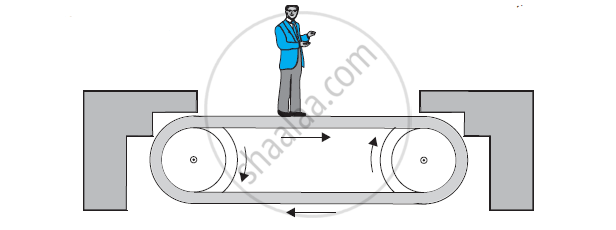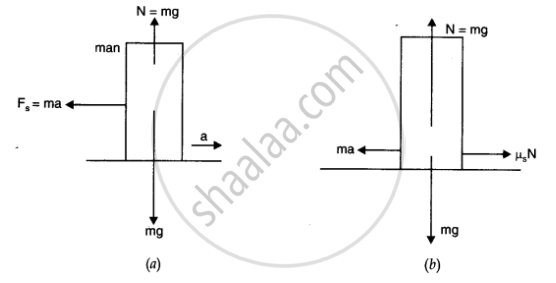CBSE (Science) Class 11CBSE
Share

# Shows a Man Standing Stationary with Respect to a Horizontal Conveyor Belt that is Accelerating with 1 M S–2. What is the Net Force on the Man? If the Coefficient of Static Friction Between the Man’S Shoes and the Belt is 0.2, up to What Acceleration of the Belt Can the Man Continue to Be Stationary Relative to the Belt - CBSE (Science) Class 11 - Physics

ConceptConservation of Momentum

#### Question

Figure  shows a man standing stationary with respect to a horizontal conveyor belt that is accelerating with 1 m s–2. What is the net force on the man? If the coefficient of static friction between the man’s shoes and the belt is 0.2, up to what acceleration of the belt can the man continue to be stationary relative to the belt? (Mass of the man = 65 kg.)#### Solution 1

Mass of the man, m = 65 kg

Acceleration of the belt, a = 1 m/s2

Coefficient of static friction, μ = 0.2

The net force F, acting on the man is given by Newton’s second law of motion as:

F_"net" = ma = 65 × 1 = 65 N

The man will continue to be stationary with respect to the conveyor belt until the net force on the man is less than or equal to the frictional force fs, exerted by the belt, i.e.,

F_"net"^' = f_s

ma^' = mu mg

a' = 0.2 × 10 = 2 m/s2

Therefore, the maximum acceleration of the belt up to which the man can stand stationary is 2 m/s2.

#### Solution 2

Here acceleration of conveyor belt a = 1 ms-2, μs= 0.2 and mass of man m = 65 kg. t As the man is in an accelerating frame, he experiences a pseudo force Fs= ma as shown in figure

Hence to maintain his equilibrium, he exerts a force F = – Fs = ma = 65 x 1 = 65 N in forward direction i.e., direction of motion of belt.

∴Net force acting on man = 65 N (forward)

As shown in fig. (b), the man can continue to be stationary with respect to belt, if force of friction

mu_s N = mu_s mg = ma_"max"

a_"max" = mu_s g = 0.2 xx 10 = 2 m s^(-2)Is there an error in this question or solution?

#### APPEARS IN

Solution Shows a Man Standing Stationary with Respect to a Horizontal Conveyor Belt that is Accelerating with 1 M S–2. What is the Net Force on the Man? If the Coefficient of Static Friction Between the Man’S Shoes and the Belt is 0.2, up to What Acceleration of the Belt Can the Man Continue to Be Stationary Relative to the Belt Concept: Conservation of Momentum.
S